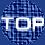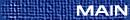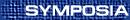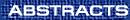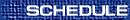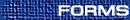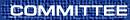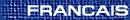CMS Krieger-Nelson Lecture / Conférence Krieger-Nelson de la SMC(Lisa Jeffrey, Organizer) LISA JEFFREY, Department of Mathematics, University of Toronto, Toronto, Ontario  M5S 3G3 Gauge theories and flat connections on Riemann surfaces Several noteworthy results in mathematics have recently been obtained by studying gauge theory on a two-dimensional spacetime (or Riemann surface); these results (formulas for correlation functions in two-dimensional Yang-Mills theory) were discovered by E. Witten (1991-92). Yang-Mills theory involves a gauge field (or connection), and the Yang-Mills action is the norm squared of its curvature. This gauge field is a generalization of the vector potential from electromagnetism, and the curvature corresponds to the tensor formed from the electric and magnetic fields. The two-dimensional case is a prototype for the four-dimensional case, and can be solved exactly. The mathematical interpretation of Witten's results is that they can be used to obtain formulas for the multiplication in the cohomology of certain moduli spaces (spaces parametrizing flat connections on Riemann surfaces). For an abelian gauge group the moduli space is a quotient of the first cohomology group of the Riemann surface. We will talk about the nonabelian case which arises in Witten's work. Witten's formulas have been given a mathematically rigorous proof (L. Jeffrey and F. Kirwan 1998) using methods from symplectic geometry, which is the natural mathematical framework for the Hamiltonian formulation of classical mechanics. On the way to outlining these results, we describe the dictionary relating the objects that appear in the physics literature and those that appear in the mathematics literature.